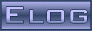Back Midas Rome Roody Rootana
 Midas DAQ System Not logged inMessage ID: 2253     Entry time: 30 Jun 2021     In reply to: 2229     Reply to this: 2265
 Author: Konstantin Olchanski Topic: Bug Fix Subject: changes in history plots
```> I am updating the history plots.
> So the idea is to use this computation:
> y_position_on_plot = offset + factor*(formula(history_value) - voffset)

Stefan and myself did some brain storming on zoom. Writing it down the way I remember it.

- we distilled the gist of the problem - the numerical values we show in the plot labels and in hover-over-the-graph
are before formula is applied or after the formula is applied?

- I suggested a universal solution using a double formula: use formula1 for one case;
use formula2 for the other case;
use formula1 for "physics calibration", use formula2 for factor and offset for composite plots:
numeric_value = formula1(history_value)
plotted_value = formula2(numeric_value)

- we agree that this is way too complicated, difficult to explain and difficult to coherently present in the history editor

- Stefan suggested a simple solution, a checkbox labeled "show raw value" next to each history variable. by default, the
value after the formula is plotted and displayed. if checked, the raw value (before the formula) is displayed, and the
value after the formula is plotted. (so this works the same as the factor and offset on the old history plots).

- if "show raw value" is enabled, the numerical values shown will be inconsistent against the labels on the vertical axis.
Our solution it to turn the axis labels off. (for composite plots, like oscillator frequency in Hz vs oscillator
temperature in degC, both scaled to see their correlation, the vertical axis is unit-less "arbitrary units", of course)

- to simplify migration of old history plots that use custom factor and offset settings, we think in the direction of
automatically moving them to the "formula". (factor=2, offset=10 automatically populates formula with "2*x+10", "show raw
value" checked/enabled). Thus we can avoid implementing factor and offset in the new history code (an unwelcome
complication).

- I think this covers all the use cases I have seen in the past, so we will move in this direction.

K.O.```
ELOG V3.1.4-2e1708b5